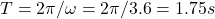## A large pendulum has a bob with mass 10 kg at the end of a thin wire of length 8 m. The bob moves in a horizontal circle at constant speed v

Question

A large pendulum has a bob with mass 10 kg at the end of a thin wire of length 8 m. The bob moves in a horizontal circle at constant speed v, with the wire making a fixed angle 18° with the vertical direction. Find the tension FT in the wire and the period T (the time for one revolution of the bob)

in progress 0
3 weeks 2021-09-05T08:15:58+00:00 1 Answers 0 views 0

FT = 103.15 N and T = 1.75 s

Explanation:

There are 3 forces at play here: gravity acting in vertical direction, tension from the string that makes 18 degrees with the vertical, and centripetal force generated by the circular motion which is horizontal.

Since the bob is moving at the constant speed, according to Newton ‘s 1st law the net force is 0. To find the tension force that makes a fixed angle of 18 degrees with the vertical, we can calculate that tension in the vertical direction and balance it with gravity force. Let g = 9.81m/s2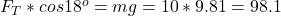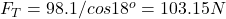To calculate the centripetal force in the horizontal direction, we can calculate tension in the horizontal direction and balance it with the centripetal force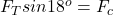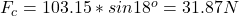The centripetal acceleration a is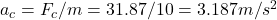The radius of circular motion is: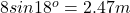The angular speed is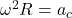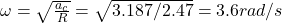The time it would take to sweep 1 period, or 2π rad is